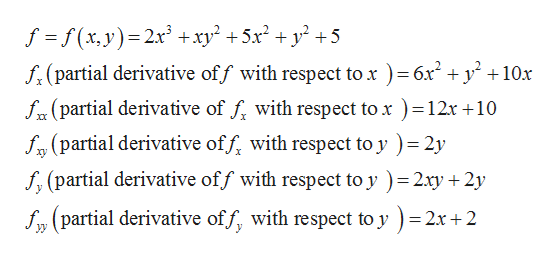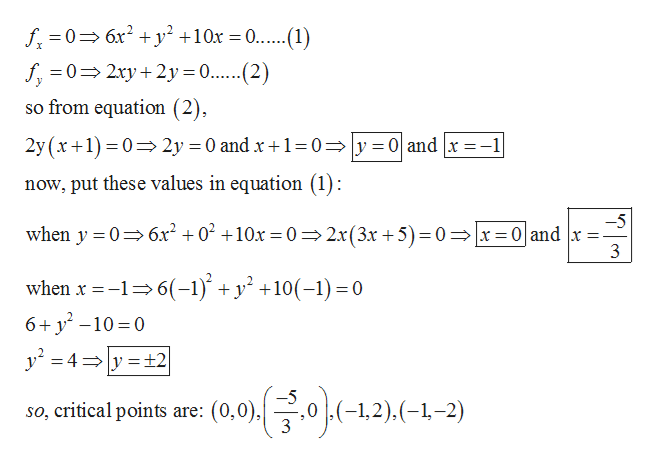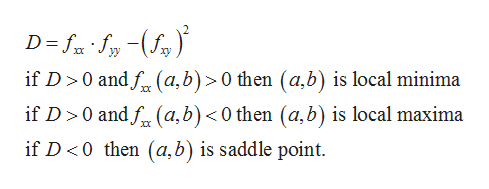# Find the local maximum and minimum values and saddle point(s) of the function. If you have three-dimensional graphing software, graph the function with a domain and viewpoint that reveal all the important aspects of the function. (Enter your answers as a comma-separated list. If an answer does not exist, enter DNE.)f(x, y) = 2x3 + xy2 + 5x2 + y2 + 5

Question
91 views

Find the local maximum and minimum values and saddle point(s) of the function. If you have three-dimensional graphing software, graph the function with a domain and viewpoint that reveal all the important aspects of the function. (Enter your answers as a comma-separated list. If an answer does not exist, enter DNE.)

f(x, y) = 2x3 + xy2 + 5x2 + y2 + 5
check_circle

Step 1

From the given information find the partial derivative:help_outlineImage Transcriptionclosef f(x,y) 2x +xy2 +5x2 + y2 +5 f(partial derivative off with respect to x ) = 6r y 10x f(partial derivative of f with respect to x ) = 12r 10 frypartial derivative off, with respect to y) 2y f(partial derivative off with respect to y ) 2xy 2y = fy partial derivative off, with respect to y 2x+2 fullscreen
Step 2

Now to find the critical points put fx and fy equal to zero:help_outlineImage Transcriptionclosef06x2y +10x = .....(1) f0 2xy2y 0..(2) so from equation (2), 2y = 0 and x +1=0y 0 and x-1 2y (x10 now, put these values in equation (1) -5 when y 0 6x2 +02 +10x = 0 2x(3x +5)= 0 x = 0 and x 3 when x -16(-1) + y +10(-1)=0 6+ y -10 0 24 y t2 -12)(--2) so, critical points are: (0,0), fullscreen
Step 3

Now write the formula for D and the conditions fo...help_outlineImage TranscriptioncloseD=S ) if D 0 and f (a, b) > 0 then (a,b) is local minima if D 0 andf (a,b) <0 then (a, b) is local maxima 2 if D <0 then (a,b) is saddle point fullscreen

### Want to see the full answer?

See Solution

#### Want to see this answer and more?

Solutions are written by subject experts who are available 24/7. Questions are typically answered within 1 hour.*

See Solution
*Response times may vary by subject and question.
Tagged in

### Math